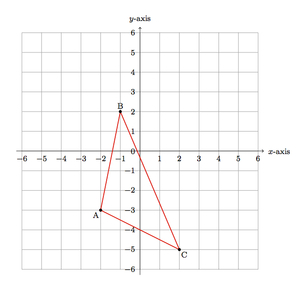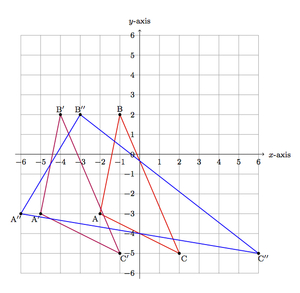# Horizontal Stretch of the Plane

Alignments to Content Standards: G-CO.A.2

Suppose $f$ is the map of the plane which takes each point $(x,y)$ to the point $(x-3,y)$ and $g$ is the map of the plane that takes each $(x,y)$ to $(3x,y)$.

1. Below is a triangle $ABC$.Show the image of $\triangle ABC$ after applying each of the maps $f$ and $g$.

2. Does $f$ preserve distances and angles? Explain.
3. Does $g$ preserve distances and angles? Explain.

## IM Commentary

The goal of this task is to compare a transformation of the plane (translation) which preserves distances and angles to a transformation of the plane (horizontal stretch) which does not preserve either distances or angles. The fact that horizontal stretch does not preserve distances can be seen from the pictures or using the Pythagorean theorem. The only line segments whose distance is preserved by this horizontal stretch are vertical lines and the teacher may wish to have students investigate this as a follow up question. The fact that horizontal stretch does not preserve angles is visibly clear but requires more careful thinking. In the solution a similarity argument is provided but students familiar with trigonometry could also calculate the angles in the different triangles and verify in this way that horizontal stretch changes the angles of this particular triangle.

The task does not specify that $f$ is a translation to the left by 3 units and that $g$ is a horizontal stretch by a factor of 3. The teacher should prompt students for a geometric description of what $f$ and $g$ do so that students understand what transformations of the plane these functions represent.

## Solution

1. Below is a picture of the images of the triangle after applying the maps $f$ and $g$:The image of $f$ is $\triangle A^\prime B^\prime C^\prime$ which is congruent to the original triangle but it is displaced to the left by 3 units. The image of $g$ is $\triangle A^{\prime \prime}B^{\prime \prime}C^{\prime \prime}$ and it is (visibly) not congruent to $\triangle ABC$.

2. The map $f$ preserves the angles of $\triangle ABC$ and more generally will preserve any angle because it is a translation: each point in the plane is displaced by the same distance in the same direction but neither distances nor angles are changed.

3. The map $g$ definitely changes distances. This is most visibly clear for $|AC|$ and $|A^{\prime \prime}C^{\prime \prime}|$. Using the Pythagorean theorem, we can check that $|AC| = \sqrt{20}$ while $|A^{\prime \prime}C^{\prime \prime}| = \sqrt{148}$. It is also clear from the picture that the angles are not congruent. For a mathematical argument, note that $|AB| = \sqrt{26}$ and $|A^{\prime \prime}B^{\prime \prime}| = \sqrt{34}$. Since there is a different scale factor between $\overline{AC}$ and $\overline{A^{\prime \prime}C^{\prime \prime}}$ on the one hand and  $\overline{AB}$ and $\overline{A^{\prime \prime}B^{\prime \prime}}$ on the other, these triangles are not similar and so their angles are not congruent.